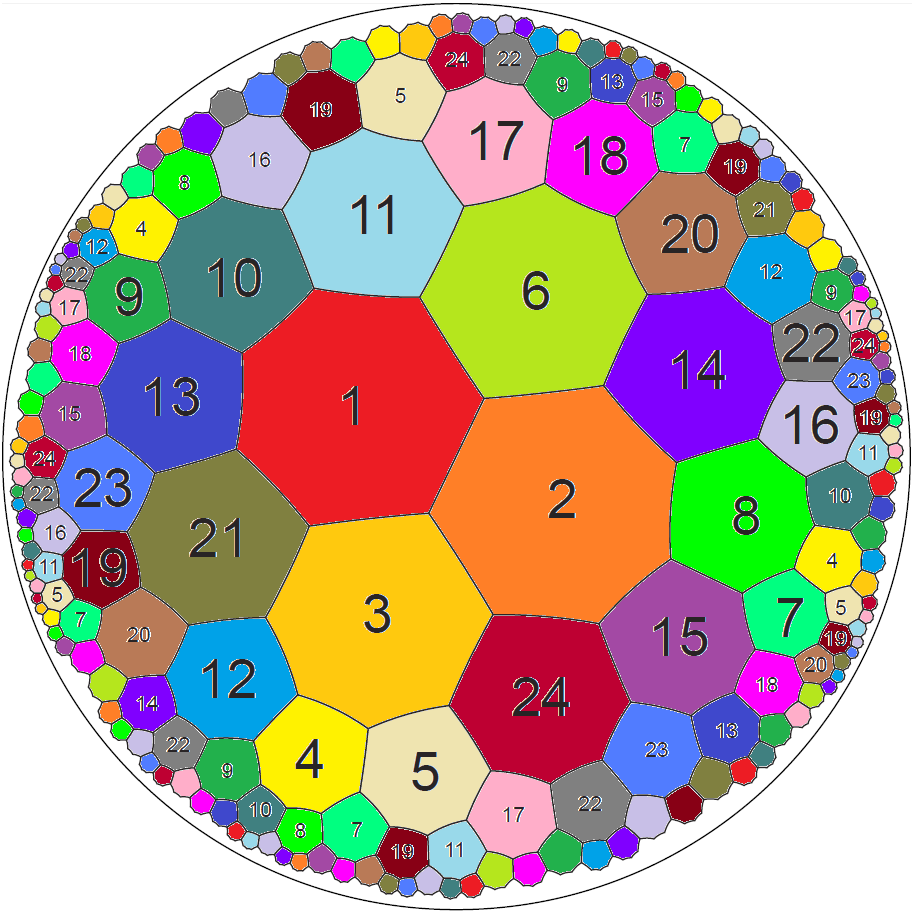Acronym heat Name hyperbolic order 3 heptagonal tiling©Circumradius sqrt[3/(12-16 cos2(π/7))] = 1.742610 i Vertex figure  Dual x3o7o Confer general polytopal classes: regular   noble polytopes Externallinks©When considering not the limit N → ∞, but rather the finite approximant N = 4, then this represents the (smallest) mod-wrap, depicted at the above right, which uses only 24 heptagons resp. 56 vertices. That one then is also known as the Klein quartic, i.e. the symmetry, which corresponds to the equation x3y + y3 + x = 0. This is why that specific mod-wrap got the Bowers acronym "quart". – To the right there is provided a faithful picture of its dual, showing up the connectivity of the 56 triangles. (Spot that it is built from red trips at the tetrahedral vertices and adjoined colorful drilled squaps at the tetrahedral edges.)

Incidence matrix according to Dynkin symbol

o3o7x   (N → ∞)

. . . | 14N |   3 |  3
------+-----+-----+---
. . x |   2 | 21N |  2
------+-----+-----+---
. o7x |   7 |   7 | 6N